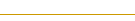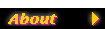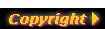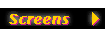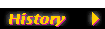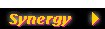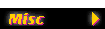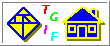William Chia-Wei Cheng (bill.cheng@usc.edu)## Tgif FAQ - eq4xpm.sym

How do I use the new eq4xpm.sym equation object?
Since tgif-4.1.42, the standard distribution of tgif comes with an additional LaTeX equation symbol file named eq4xpm.sym. This file is similar to eq4.sym but offers an alternative to make an equation in tgif. Instead of an EPS file an XPM image is created. This is usefull if one wants to have the equation visible in tgif, and especially in the slide-show mode.

The use of this alternative symbol file is, however, only possible, if one has LaTeX, ghostscript, convert from ImageMagic, the netpbm package, and eventually xv. Xv is only necessary, if editing the raster image is desired (see proc= attribute).

Below is a description about how to use this symbol file.

Make sure that latex, dvips, convert (ImageMagic) and the netpbm package as well as xv (eventually) are in your program path. After you instantiate this symbol, you can change the resolution density and the geometry in order to enhance the quality. To do this, right-click on the symbol and choose "Edit Attribute in Editor". Ther you can find the density= and the geometry= Attributes. The default values already give quite a reasonable quality of the equation image. Under this menue, you can also find the eq= Attribute, where you can edit the equation in TeX-style. As soon as the symbol is double-clicked for the first time, you will be prompted for an equation name, which you just can answer with <ENTER>, if you don't need to refer to the equation. The equation name will then be a unique number.

After this the LaTeX scripts will run as well as the image conversions. If the proc= attribute contains the string cmd, then the image will directly appear in tgif. If it contains the string ecmd, then xv will be launched before inclusion of the equation image. You then have the possibility to manipulate the image. Please note that you have to save the image then as XPM and quit xv in order to include the image in tgif. The eq= attribute visible in the beginning is hidden after the first compilation.

How do I get the eq4xpm.sym equation object to make a better looking image?
If you right-click on the eq4xpm.sym object and select Edit Attribute in Editor and select "cmd=", you will see the following lines:
```    cd /tmp;
xterm -geom 80x8+300+100 -e latex \$(file_name);
xterm -geom 80x8+300+100 -e dvips -E -n 1 -o \$(file_name).ps \$(file_name);
xterm -geom 80x8+300+100 -e convert -geometry \$(geometry) \
-density \$(density) -bordercolor white -border 0 \$(file_name).ps \$(file_name).gif;
giftopnm \$(file_name).gif | ppmtoxpm > \$(file_name).xpm;```

Removing the "xterm" part of the command, you get:

```    cd /tmp;
latex \$(file_name);
dvips -E -n 1 -o \$(file_name).ps \$(file_name);
convert -geometry \$(geometry) -density \$(density) \
-bordercolor white -border 0 \$(file_name).ps \$(file_name).gif;
giftopnm \$(file_name).gif | ppmtoxpm > \$(file_name).xpm;```

These are basically the commmands tgif will execute to produce the XPM file which tgif will import into the equation object. The values for \$(geometry) and \$(density) are stored in attributes of the eq4xpm.sym object and they are, by default, 25% and 576, respectively. You can change these values to get a larger equation. For example, if you change the value of the geometry= attribute to 100%, the image will be 4 times as large as before.

Another thing you can do is to use the Times font which has a better resolution than the Computer Modern font of LaTeX. To get this effect, you need to modify the latex source to include \usepackage{times}. Right-click on the eq4xpm.sym object and select Edit Attribute in Editor and select header=. Add the following right below the \documentclass[12pt]{article} line:

`    \usepackage{times}`

Save the file and exits, then double-click the equation object again.

If my file contains a eq4xpm.sym equation object and I export the file in the PS/EPS format, the quality of the equation looks pretty bad. How can I fix this?
The eq4xpm.sym object generates a bitmap file, so it doesn't look very good in PS/EPS unless you generate a very large bitmap. To get a large bitmap, please see the above section. If you need to generate EPS file, you should use eq4.sym.